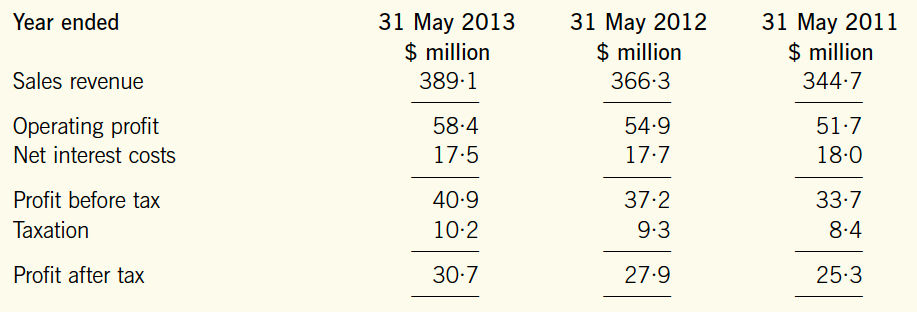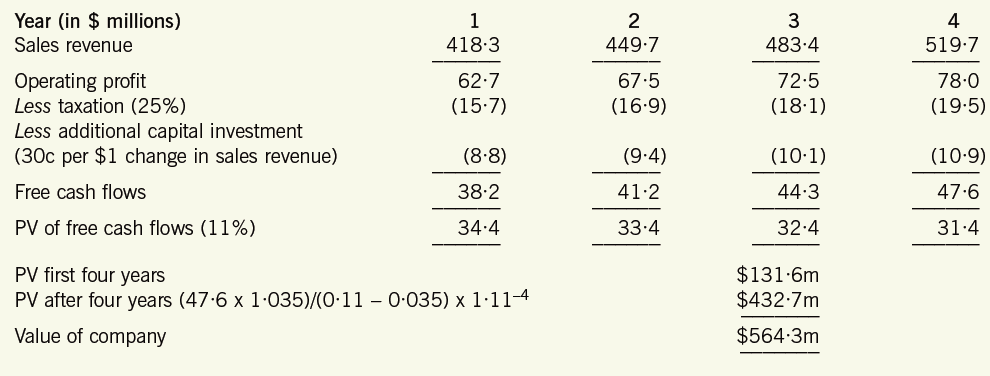AFMP4
Syllabus B. Advanced Investment Appraisal B4. Valuation and the use of free cash flows

# B4a/C2b. Discounted Free cash flow basis 8 / 11

### Syllabus B4a/C2b)

B4a) Apply asset based, income based and cash flow based models to value equity. Apply appropriate models, including term structure of interest rates, the yield curve and credit spreads, to value corporate debt.

C2b) Estimate the potential near-term and continuing growth levels of a corporation’s earnings using both internal and external measures.

### This method is based upon the PV of the free cash flow to equity of an enterprise.

#### Free cash flow to equity is the cash flow available to a company from operations after:

1. interest expenses

2. tax

3. repayment of debt and lease obligations

4. any changes in working capital

5. capital spending on assets needed to continue existing operations (ie replacement capital expenditure equivalent to economic depreciation)

#### Remember!

Discounted FCF is used for the calculation of the Value of Company attributable to equity holders.

Value of Company = PV of Free cash flows (FCF)

#### How to calculate the PV of FCF using a CONSTANT annual growth rate

e.g. After four years, the annual growth rate of the FCF to the company will be 3%, for the foreseeable future.

FCF in Y4 = 100
g = 3%
k = 11%
PV of FCF (first 4 years) 500

• PV of FCF, year 5 onwards
= [FCF (in Y4) x (1 + growth rate (g))  /  (cost of capital (k) – g) ] x (1+k) (to the  negative power of the number of years before the g is consistent each year)

= (100 x 1.03) / (0.11 - 0.03)] x 1.11 ^ - 4
= 1,287.5 x 0.6587
= 848

#### Value of Company

• = PV of FCF (first 4 years) + PV of FCF, year 5 onwards

= 500 + 848
= 1,348

#### Example

COW Co’s future sales revenue will increase by 7.5% for the next four years.

After the four years, the annual growth rate of the free cash flows to the company will be 3.5%, for the foreseeable future.

Operating profit margins are expected to be 15% in the future.

Although it can be assumed that the current tax-allowable depreciation is equivalent to the amount of investment needed to maintain the current level of operations, the company will require an additional investment in assets of 30c per \$1 increase in sales revenue for the next four years.

Tax rate is 25%.

Cost of capital is 11%.

Extract from COW Co's Statement of profit or loss:#### Solution

Sales revenue in Y1 = \$389.1 x 1.075 = \$418.3
Operating profit in Y1 = \$418.3 x 15% = \$62.7
Additional capital investment in Y1 = (\$418.3 - \$389.1) x \$0.30 = \$8.8